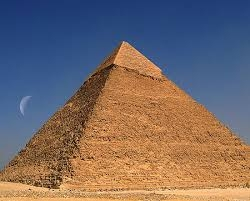# The pyramid

The pyramid with a square base is 50 m high and the height of the sidewall is 80 m. Find the endge of the base of the pyramid.

a =  124.9 m

### Step-by-step explanation:Did you find an error or inaccuracy? Feel free to write us. Thank you!Tips to related online calculators
Do you want to convert length units?
Pythagorean theorem is the base for the right triangle calculator.

#### You need to know the following knowledge to solve this word math problem:

We encourage you to watch this tutorial video on this math problem:

## Related math problems and questions:

• Tetrahedral pyramidIt is given a regular tetrahedral pyramid with a base edge of 6 cm and the height of the pyramid 10 cm. Calculate the length of its side edges.
• Height of pyramidThe pyramid ABCDV has edge lengths: AB = 4, AV = 7. What is its height?
• Rectangular base pyramidCalculate an area of the shell of the pyramid with a rectangular base of 2.8 m and 1.4 m and height 2.5 meters.
• Regular 4-sided pyramidFind the area (surface area) of a regular 4-sided pyramid if its height is 20 m and the wall height is 23 m.
• Hexagonal pyramidPlease calculate the height of a regular hexagonal pyramid with a base edge of 5cm and a wall height of w = 20cm. Please sketch a picture.
• Hexagonal pyramidCalculate the volume and surface area of a regular hexagonal pyramid with a base edge a = 30 m and a side edge b = 50 m.
• The pyramid 4sThe pyramid with a rectangular base measuring 6 dm and 8 dm has a side edge of length 13 dm. Calculate the surface area and volume of this pyramid.The regular quadrilateral pyramid has a base edge a = 1.56 dm and a height h = 2.05 dm. Calculate: a) the deviation angle of the sidewall plane from the base plane b) deviation angle of the side edge from the plane of the base
• SquareCalculate the area of the square shape of the isosceles triangle with the arms 50m and the base 60m. How many tiles are used to pave the square if the area of one tile is 25 dm2?
• Four sided prismCalculate the volume and surface area of a regular quadrangular prism whose height is 28.6cm and the body diagonal forms a 50-degree angle with the base plane.
• The tentCalculate how much cover (without a floor) is used to make a tent that has the shape of a regular square pyramid. The edge of the base is 3 m long and the height of the tent is 2 m.
• DitchDitch profile is an isosceles trapezoid with bases of length 80m and 60m. The slope of the side wall of the ditch is 80°. Calculate the ditch depth.
• Body diagonalCuboid with base 7cm x 3,9cm and body diagonal 9cm long. Find the height of the cuboid and the length of the diagonal of the base,
• PyramidThe pyramid has a base rectangle with a = 6cm, b = 8cm. The side edges are the same and their length = 12.5 cm. Calculate the surface of the pyramid.
• Square pyramidCalculate the pyramid's volume with the side 5cm long and with a square base, side-base has an angle of 60 degrees.
• How toHow to find a total surface of a rectangular pyramid if each face is to be 8 dm high and the base is 10 dm by 6 dm.
• Pyramid roof2/4 of the area of ​​the roof-shaped regular tetrahedral pyramid with base edge 10 m and height of 4 m is already covered with roofing. How many square meters still need to be covered?